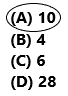# Texas Go Math Grade 3 Lesson 12.4 Answer Key Divide by 3

Refer to our Texas Go Math Grade 3 Answer Key Pdf to score good marks in the exams. Test yourself by practicing the problems from Texas Go Math Grade 3 Lesson 12.4 Answer Key Divide by 3.

## Texas Go Math Grade 3 Lesson 12.4 Answer Key Divide by 3

Essential Question
What strategies can you use to divide by 3?

Unlock the Problem
For field day, 18 students have signed up for the relay race. Each relay team needs 3 students. How many teams can be made?

• What do you need to find?
The number of teams needs to find.

• Circle the numbers you need to use.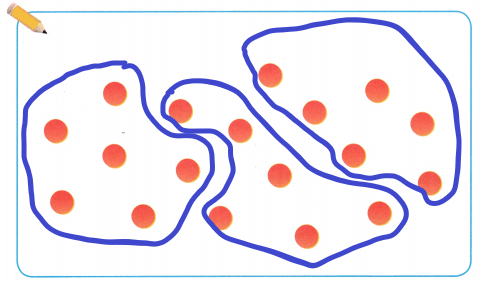One Way
Make equal groups.

• Look at the 18 counters below.
• Circle as many groups of 3 as you can.
• Count the number of groups.There are _6_ groups of 3.
So, __6_ teams can be made.
You can write 18 ÷ 3 = _6_ or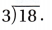Math Talk
Mathematical processes
Suppose the question asked how many students would be on 3 equal teams. How would you model 3 equal teams? Would the quotient be the same?

Another Way
Use a related multiplication fact.
Since division is the opposite of multiplication, think of a related multiplication fact to find 18 ÷ 3.Share and ShowQuestion 1.
Circle groups of 3 to find 12 ÷ 3. ___Explanation:There are 12 counters and 4 circles.
A Group of 3 counters should be circled so we got 4 circles. And if we divide 12/3 then also we get 4.

Math Talk
Mathematical Processes
Explain what the number of circles you made tells you.

Find the quotient.

Question 2.
6 ÷ 3 = ___
Explanation:Here I placed 6 counters and 2 groups, each group contain 3 counters.
Question 3.
___ = 14 ÷ 2
Explanation: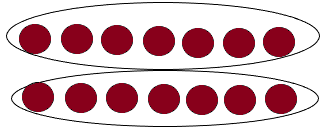Here I placed 14 counters and 2 groups, each group containing 7 counters.
Therefore, 14/2=7.

Question 4.
21 ÷ 3 = ___
Explanation: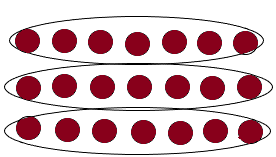Here I placed 21 counters and I divided them into 3 groups, each group containing 7 counters.
Therefore, 21/3=7.

Question 5.
__ = 30 ÷ 5
Explanation:Here I placed 30 counters and I made 5 groups, each group containing 6 counters.
Therefore, 30/5=6.
Problem Solving

Practice: Copy and Solve Find the quotient. Draw a quick picture to help.

Question 6.
9 ÷ 3
Explanation: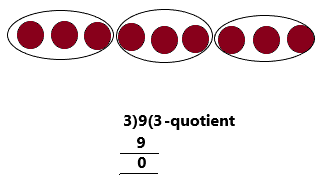From the diagram, I placed 9 counters and made 3 groups, each group containing 3 counters.

Therefore, 9/3=3. 3 is the quotient.

Question 7.
10 ÷ 5
Explanation: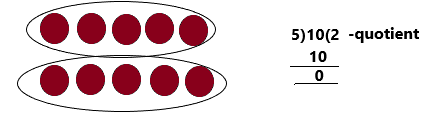From the diagram, I placed 10 counters and made 2 groups, each group containing 5 counters.
Therefore, 10/5=2. 2 is the quotient.

Question 8.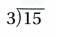Explanation:
3√15= 3√5*3            √ab=√a*√b
=3√5*√3                   (value of √5=2.2361 and √3=1.7321)
=3(2.2361)(1.7321)
after multiplying the above step, then we get
=11.61.
Another way
3√15=3(3.8730)               (value of √15=3.8370)
=11.51

Question 9.Explanation:
=3.√9*2            {square root of 9 is 3}.
=3(3)√2
=9√2.

H.O.T. Algebra Write +, x, or ÷.

Question 10.
25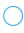5 = 10 ÷ 2
Explanation:
The division symbol is correct.
25÷5=10÷2
After simplifying this we get
5=5.
Question 11.
3 × 3 = 63
Explanation:
If we write the ‘+’ symbol then both equations will be equal.
3×3=6+3
9=9.

Question 12.
162 = 24 – 16
Explanation:
If we write the ‘÷’ symbol then both equations will be equal.
16÷2=24-16
8=8.

Question 13.
13 + 19 = 84
Explanation:
If we write the ‘×’ symbol then both equations will be equal.
13+19=8×4
32=32.

Question 14.
142 = 16 × 2
Explanation:
If we write the ‘×’, ‘+’, ‘÷’ symbol. then the given equation will be not equal.
14×2=16×2
14+2=16×2
14÷2=16×2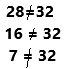Question 15.
21 ÷ 3 = 52
Explanation:
If we write the ‘+’ symbol then both equations will be equal.
21÷3=5+2
7=7.

Question 16.
There are 7 equal teams for the relay race. There are 21 students that have signed up for the relay race. How many students are on each team?
Explanation:
The total number of students signed up for the relay race=21.
The number of equal teams for the relay race=7.
The number of students is on each team=21/7=3.
Therefore, 3 students on each team.

Problem Solving

Use the table for 17-18.

Question 17.
There are 5 equal teams in the relay race. How many students are on each team? Write a division equation that shows the number of students on each team.Explanation:
In the given table, for the relay race activity, 25 students are present. In the question also they have asked for relay race only. So we should take relay race students only.
Now in the question already given that there are 5 equal teams in this activity. We need to find out the number of students is there in each group. And moreover, the division equation could be 25÷5.
After simplifying the equation we will get 5.
Therefore, 5 students in each group.

Question 18.
H.O.T. Multi-Step Students doing the jump-rope race and the beanbag toss compete in teams of 3. How many more teams participate in the jump-rope race than in the beanbag toss? Explain how you know.First, we write the number of students involved in the jump-rope race is 27 and the beanbag toss activity is 18. They are competing in teams of 3.
beanbag toss teams=18÷3=6.
jump rope race teams=27÷3=9.
Here we need to find out the number of teams participating in the jump rope race than in beanbag toss=9-6=3.
Therefore, 3 more teams are participating in the jump-rope race than in the beanbag toss.

Question 19.
H.O.T. What’s the Question? Michael puts 21 sports cards into stacks of 3. The answer is 7 stacks.
Explanation:
The total number of sports cards=21
He arranged stacks with number of cards=3
The number of stacks he arranged=21÷3=7.
Question 20.
Communicate Olivia buys 24 beanbags for field day. She buys 3 equal packs. Write a related multiplication fact to find the number of beanbags in each pack?
Explanation:
The number of bags Olivia buys=24.
The number of equal packs she buys=3
We need to find out the number of beanbags in each pack=24÷3=8.
Here, the multiplication factor asked so, it could be 3×8=24, and 8×3=24.

Fill in the bubble for the correct answer choice.

Question 21.
Sam puts a total of 15 biscuits into the feeder for 3 giraffes at the San Diego Zoo. Each giraffe eats the same number of biscuits. Which shows the related multiplication fact?
(A) 12 = 3 × 4
(B) 15 ÷ 3 = 5
(C) 3 × 5 = 15
(D) 15 ÷ 5 = 3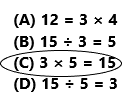Explanation:
Total number of biscuits sam puts into the feeder=15

The number of girafees=3
Question 22.
Carlos pays $24 for 3 comic books. Each comic book costs the same amount. How much does each comic book cost? (A)$8
(B) $27 (C)$7
(D) $9 Answer: Option A is correct.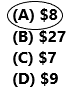Explanation: Total amount Carlos pays for books=24$

The number of books he pays=3
Cost of each cosmic book=24÷3=8.
Therefore, each book costs 8$. Question 23. Multi-Step Sue plants 18 pink flowers and 9 yellow flowers in flowerpots. She plants 3 flowers in each flowerpot. How many flowerpots does Sue use? (A) 15 (B) 3 (C) 8 (D) 9 Answer: Option D is correct.Explanation: The total number of flowers Sue plants=18+9=27. The number of flowers she plants in each pot=3 The number of flowerpots Sue use=27÷3=9. Therefore, she uses 9 flowerpots. Texas Test Prep Question 24. Apply Miguel planted 21 flowers in 3 rows. How many flowers are in each row? (A) 24 (B) 8 (C) 7 (D) 18 Answer: Option C is correct.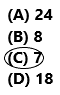Explanation: The number of flowers planted by Miguel=21 The number of rows he applied=3 The number of flowers in each row=21÷3=7. Therefore, 7 flowers in each row. ### Texas Go Math Grade 3 Lesson 12.4 Homework and Practice Answer Key Circle groups of 3. Question 1. Find 15 ÷ 3.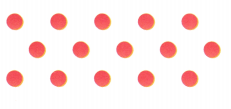Answer: 5 Explanation: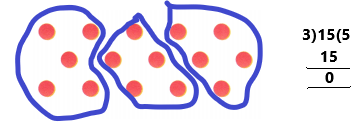For the given 15 counters and I made a group of 3. In that each group there are 5 counters placed. Find the quotient. Question 2. Find 9 ÷ 3.Answer: 3 Explanation:For the given 9 counters and I made a group of 3. In that each group there are 3 counters placed. Find the quotient. Question 3. 18 ÷ 3 = ___ Answer: 6 Explanation: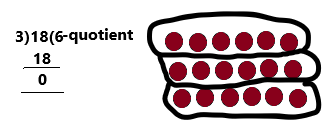I had placed 18 counters and I made a group of 3. In that each group there are 6 counters placed. The quotient is 6. Question 4. ___ = 27 ÷ 3 Answer: 9 Explanation: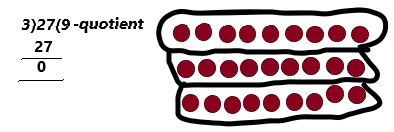I had placed 27 counters and I made a group of 3. In that each group there are 9 counters placed. The quotient is 9. Question 5. 24 ÷ 3 = ____ Answer: 8 Explanation:I had placed 24 counters and I made a group of 3. In that each group there are 8 counters placed. The quotient is 8. Problem Solving Use the table for 6-8. Question 6. Granola bars are in equal groups on Food 3 trays. Write a division equation that shows how many granola bars are on each tray.Answer: 9 Explanation: The total number of granola bars=27{given in the table}. The number of trays of equal groups =3 The number of granola bars in each tray=27÷3=9. Therefore, 9 granola bars in each tray. Question 7. Muffins and apple tarts are each served on trays in equal groups of 3. How many more trays are needed for the apple tarts than the muffins? Explain. Answer: 3 Explanation: The number of Muffins=15 The number of apples tarts=24 Both are served on trays in equal groups=3 Muffins served on each tray=15÷3=5 apple tarts served on each tray=24÷3=8 Now we need to find out the number of trays is needed for the apple tarts than the muffin=8-5=3. Therefore, 3 more trays are needed. Question 8. What if 9 more muffins were added to the Food Fair Snacks table? How many muffins would be on 3 trays? Answer: 8 Explanation: The number of muffins in the given table=15 If we add 9 more muffins which means 15+9=24. Now we have a total of 24 muffins. Here, we need to find out the number of muffins would be on 3 trays=24÷3=8. Therefore, 8 muffins would be there. Texas Test Prep Fill in the bubble completely to show your answer. Question 9. There are 18 students in music class. The teacher puts the students into 3 equal groups. How many students are in each group? (A) 5 (B) 15 (C) 6 (D) 21 Answer: Option C is correct.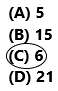Explanation: The total number of students in music class=18 The number of equal groups the teacher puts=3 The number of students in each group=18÷3=6. Therefore, 6 students in each group. Question 10. Mr. Martin has 30 desks in his classroom. He arranges the desks into 3 equal rows. How many desks are in each row? (A) 27 (B) 33 (C) 1o (D) 15 Answer: Option C is correct.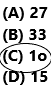Explanation: The total number of desks in his classroom=30 The number of rows he arranged equally=3 The number of desks in each row=30÷3=10. Therefore, 10 desks in each row. Question 11. Kim pays$15 for 3 boxes of cat food. Each box costs the same amount. How much does each box of cat food cost?
(A) $12 (B)$5
(C) $3 (D)$4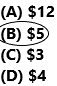Explanation:
The amount Kim pays for cat food=15$. The number of boxes he pays=3 The cost of each box of cat food=15÷3=5$.
Therefore, 5\$ is the box cost.

Question 12.
Cho makes 12 waffles. He puts an equal number of waffles on 3 plates. How many waffles are on each plate?
(A) 9
(B) 15
(C) 5
(D) 4Explanation:
The number of waffles Cho makes=12
The number of plates he puts equally=3
The number of waffles is on each plate=12÷3=4.
Therefore, 4 waffles on each plate.

Question 13.
Multi-Step Jem pastes 21 photos and 15 postcards in a scrap album. She puts 3 on each page. How many pages does Jem fill in the scrap album?
(A) 7
(B) 5
(C) 12
(D) 6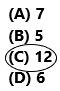Explanation:
The number of postcards and photos are posted in the scrap album=21+15=36.
The number of things she used to put on each page=3
The number of pages Jem fill in the scrap album=36÷3=12.
Therefore, Jem fills 12 pages.

Question 14.
Multi-Step Blaine makes an array of 12 red squares and 18 blue squares. She puts 3 squares in each row. How many rows does Blaine have in the array?
(A) 10
(B) 4
(C) 6
(D) 28